Miscellaneous

Chapter 4 Class 12 Determinants
Serial order wise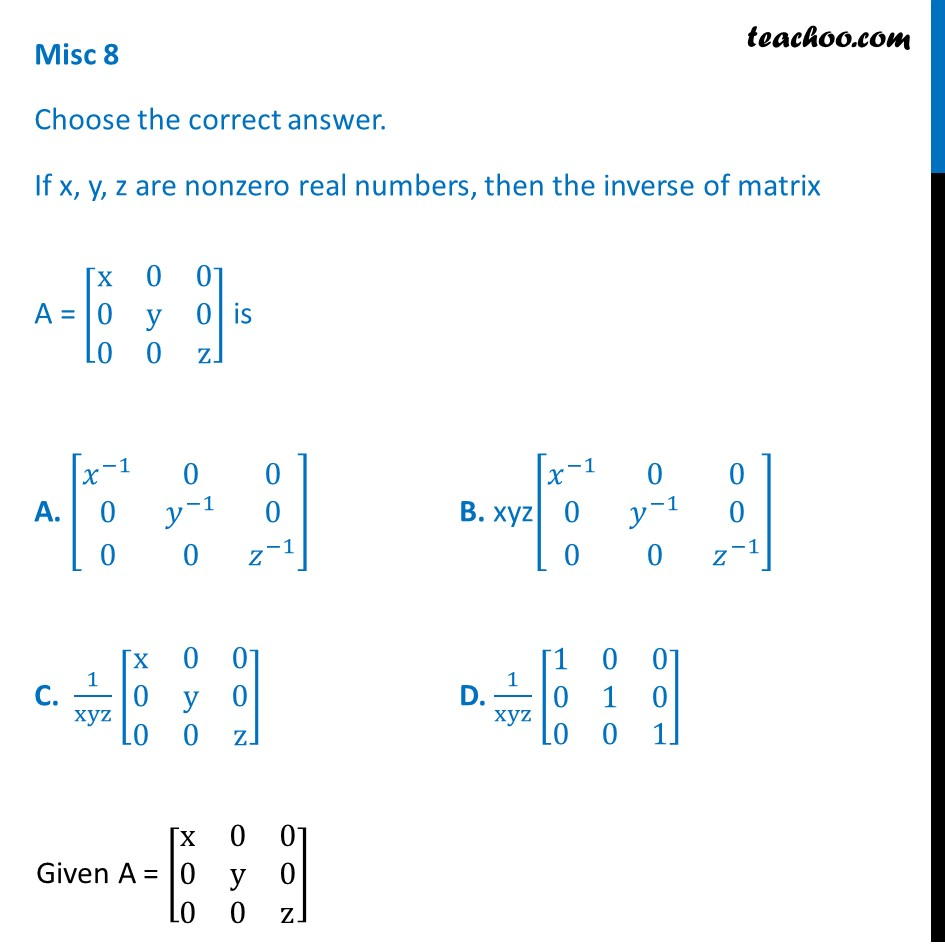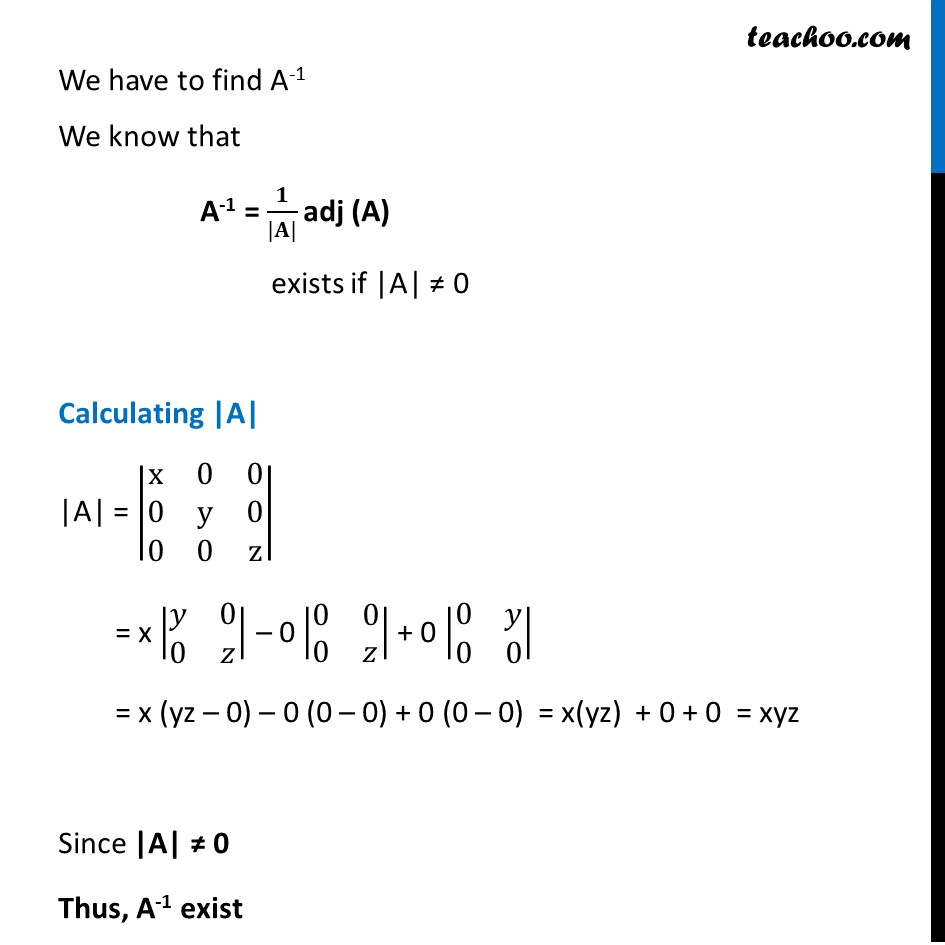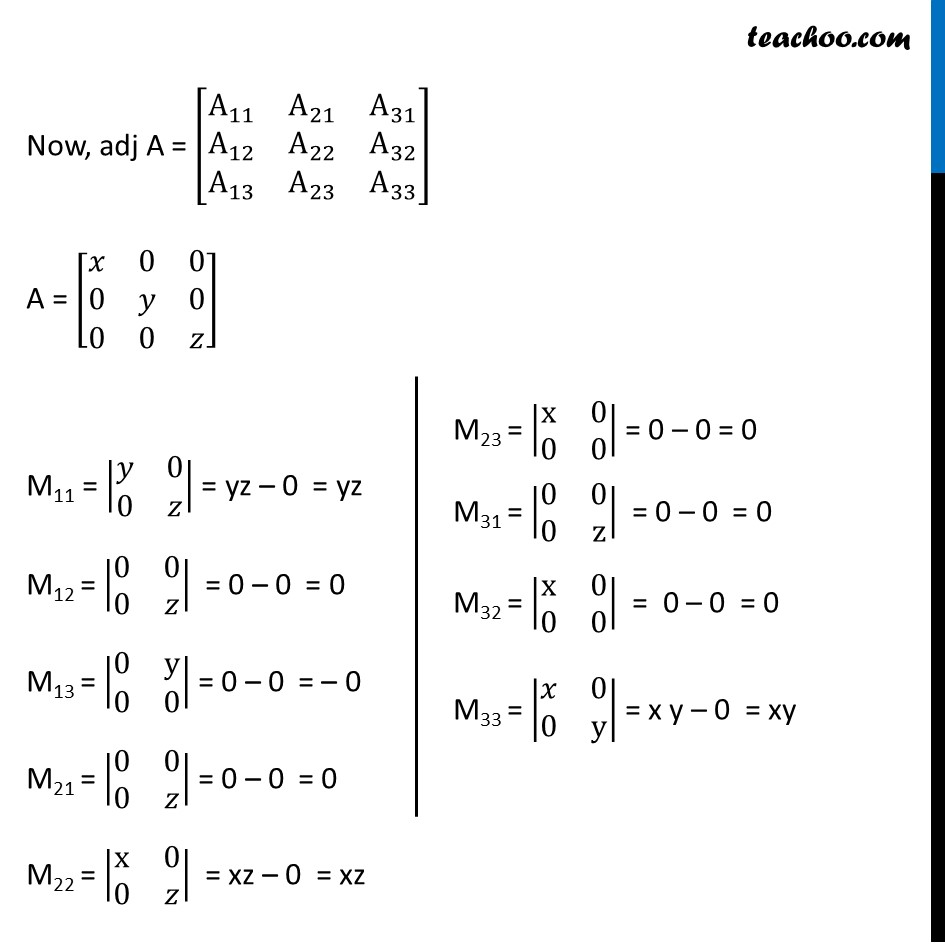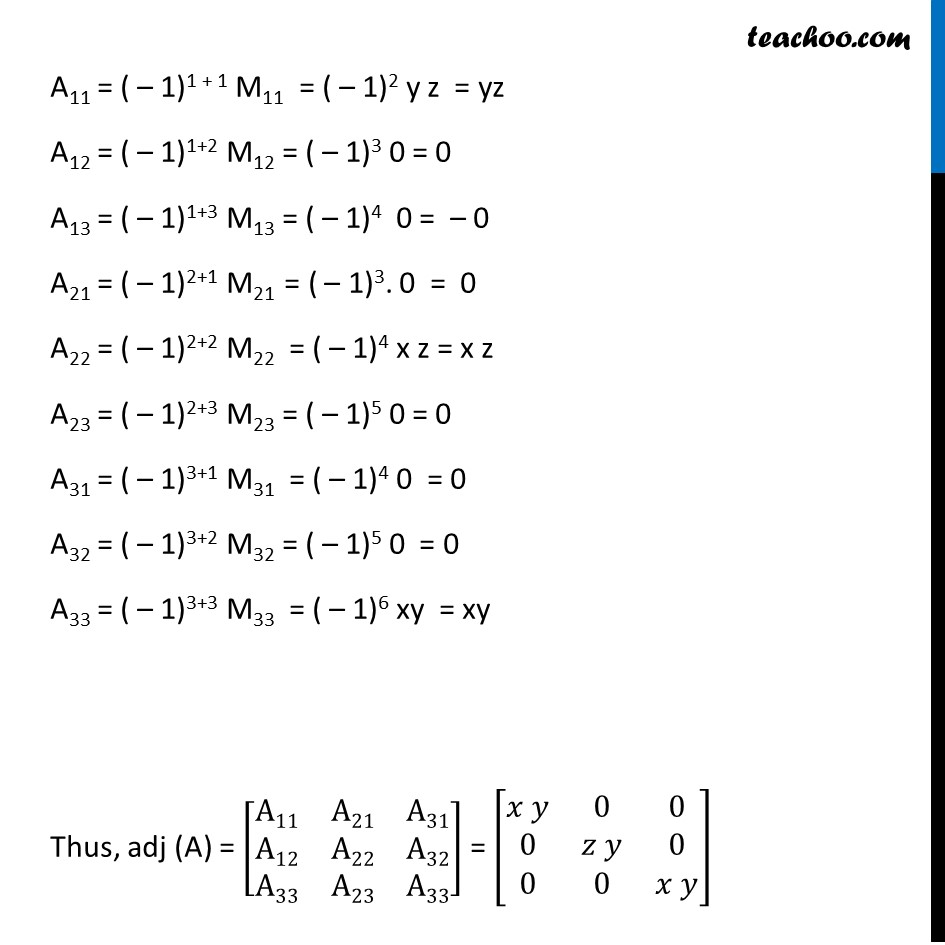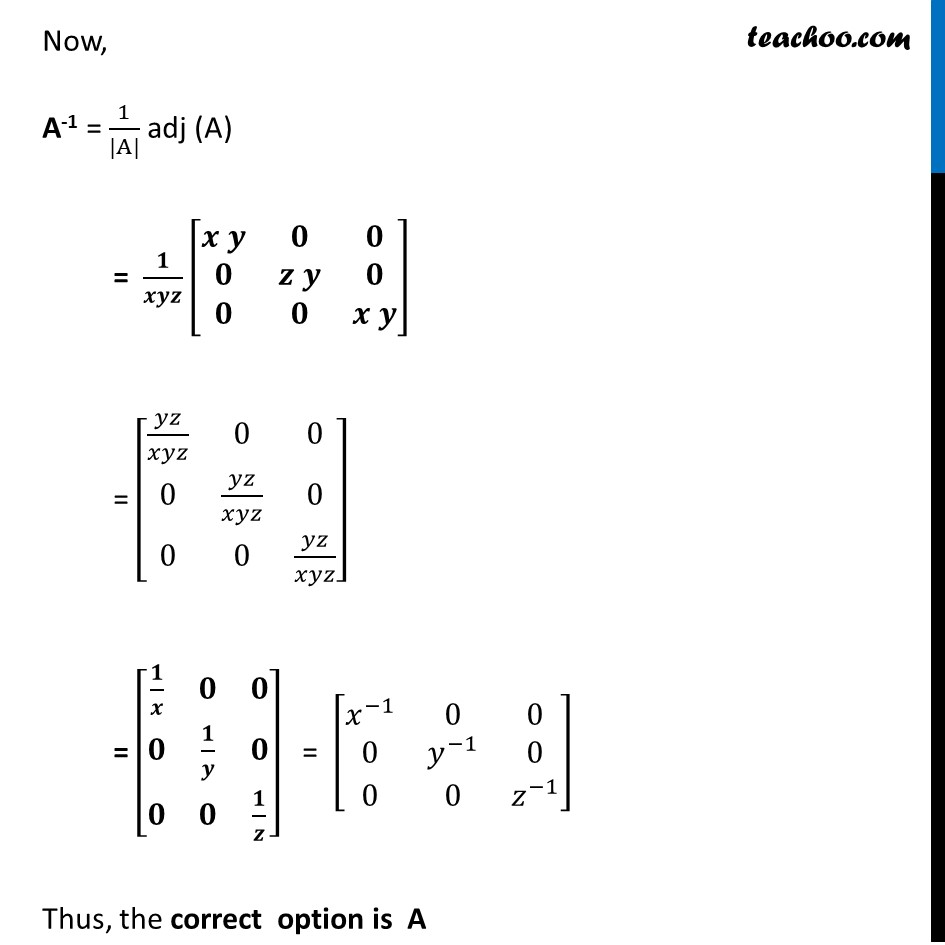Learn in your speed, with individual attention - Teachoo Maths 1-on-1 Class

### Transcript

Misc 8 Choose the correct answer. If x, y, z are nonzero real numbers, then the inverse of matrix A = [■8(x&0&0@0&y&0@0&0&z)] is A. [■8(𝑥^(−1)&0&0@0&𝑦^(−1)&0@0&0&𝑧^(−1) )] B. xyz[■8(𝑥^(−1)&0&0@0&𝑦^(−1)&0@0&0&𝑧^(−1) )] C. 1/xyz [■8(x&0&0@0&y&0@0&0&z)] D. 1/xyz [■8(1&0&0@0&1&0@0&0&1)] Given A = [■8(x&0&0@0&y&0@0&0&z)] We have to find A-1 We know that A-1 = 𝟏/(|𝐀|) adj (A) exists if |A| ≠ 0 Calculating |A| |A| = |■8(x&0&0@0&y&0@0&0&z)| = x |■8(𝑦&0@0&𝑧)| – 0 |■8(0&0@0&𝑧)| + 0 |■8(0&𝑦@0&0)| = x (yz – 0) – 0 (0 – 0) + 0 (0 – 0) = x(yz) + 0 + 0 = xyz Since |A| ≠ 0 Thus, A-1 exist Now, adj A = [■8(A_11&A_21&A_31@A_12&A_22&A_32@A_13&A_23&A_33 )] A = [■8(𝑥&0&0@0&𝑦&0@0&0&𝑧)] M11 = |■8(𝑦&0@0&𝑧)| = yz – 0 = yz M12 = |■8(0&0@0&𝑧)| = 0 – 0 = 0 M13 = |■8(0&y@0&0)| = 0 – 0 = – 0 M21 = |■8(0&0@0&𝑧)| = 0 – 0 = 0 M22 = |■8(x&0@0&𝑧)| = xz – 0 = xz M23 = |■8(x&0@0&0)| = 0 – 0 = 0 M31 = |■8(0&0@0&z)| = 0 – 0 = 0 M32 = |■8(x&0@0&0)| = 0 – 0 = 0 M33 = |■8(𝑥&0@0&y)| = x y – 0 = xy A11 = ( – 1)1 + 1 M11 = ( – 1)2 y z = yz A12 = ( – 1)1+2 M12 = ( – 1)3 0 = 0 A13 = ( – 1)1+3 M13 = ( – 1)4 0 = – 0 A21 = ( – 1)2+1 M21 = ( – 1)3. 0 = 0 A22 = ( – 1)2+2 M22 = ( – 1)4 x z = x z A23 = ( – 1)2+3 M23 = ( – 1)5 0 = 0 A31 = ( – 1)3+1 M31 = ( – 1)4 0 = 0 A32 = ( – 1)3+2 M32 = ( – 1)5 0 = 0 A33 = ( – 1)3+3 M33 = ( – 1)6 xy = xy Thus, adj (A) = [■8(A11&A21&A31@A12&A22&A32@A33&A23&A33)] = [■8(𝑥 𝑦&0&0@0&𝑧 𝑦&0@0&0&𝑥 𝑦)] Now, A-1 = 1/(|A|) adj (A) = 𝟏/𝒙𝒚𝒛 [■8(𝒙 𝒚&𝟎&𝟎@𝟎&𝒛 𝒚&𝟎@𝟎&𝟎&𝒙 𝒚)] = [■8(𝑦𝑧/𝑥𝑦𝑧&0&0@0&𝑦𝑧/𝑥𝑦𝑧&0@0&0&𝑦𝑧/𝑥𝑦𝑧)] = [■8(𝟏/𝒙&𝟎&𝟎@𝟎&𝟏/𝒚&𝟎@𝟎&𝟎&𝟏/𝒛)] = [■8(𝑥^(−1)&0&0@0&𝑦^(−1)&0@0&0&𝑧^(−1) )] Thus, the correct option is A